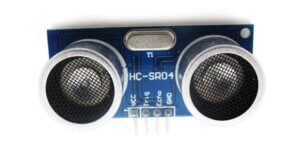Raspberry Pi Projects

# Raspberry Pi With Ultrasonic Sensor Tutorial

This is a tutorial on Raspberry Pi With Ultrasonic Sensor Tutorial or how to use an ultrasonic sensor module with a Raspberry Pi. The ultrasonic sensor module is used to measure distance. The module can be used to measure the distance to an object. The module can also be used to detect ultrasonic sounds.

## The HC-SR04 moduleThe HC-SR04 module is an Ultrasonic sensor that can be used with Raspberry Pi or Arduino. It is a cheap and easy way to get started with using Ultrasonic sensors. The sensor can be used to measure distance, and can also be used to detect objects.

The sensor has two transducers, one that emits a sound wave, and one that receives the sound wave. The sound wave is used to determine the distance to an object. The HC-SR04 module can be used to measure distances up to 400 cm. The module is easy to use and can be interfaced with Raspberry Pi using just a few lines of code.

### The HC-SR04 pinout

The HC-SR04 pinout is as follows:• Vcc is the power supply voltage, which is +5V for the HC-SR04.
• Trig is the trigger input,
• The echo is the echo output.
• GND is the ground.

## How Does the Ultrasonic Sensor Work?The HC-SR04 sensor works by sending out a ray of ultrasound at a frequency of 40kHz. When this ultrasound hits an object, it will bounce back and be received by the HC-SR04. The HC-SR04 will then output a pulse whose width is proportional to the time it took for the ultrasound to bounce back. The HC-SR04 can be used to measure distance in a variety of applications. For example, it could be used to measure the distance between a sensor and an object in order to determine if the object is close enough to trigger a reaction.

`Distance = Time x Speed`

• Time – Time that is taken by the sound wave to reach the receiver
• Speed – Speed of the sound wave.

Interesting Note –

1. Speed of sound changes due to the change in temperature and change in humidity.

2. The speed of sound, however, is 4.3 times as fast in water as in air

## Connecting The Raspberry Pi With Ultrasonic Sensor

As discussed before, ultrasonic sensors have a transmitter (Trigger) that can be operated to transmit infrared sound waves and have a receiver that receives reflected sound waves(ECHO pin).The trig pin must be connected to an output pin, and the echo pin must be connected to an input pin. Then, the microcontroller can be used to trigger the sensor and read the echo pulse. The HC-SR04 is a viral sensor and is relatively easy to use. It is a versatile sensor that can

## Python Script for Raspberry Pi With Ultrasonic Sensor

Now for the script to really take some measures. In this example, I am using Python. Why Python? It’s a famous language on the Raspberry Pi.

```# Measure distance using an ultrasonic module

# -----------------------
# Import required Python libraries
# -----------------------
import time
import RPi.GPIO as GPIO

# -----------------------
# Define some functions
# -----------------------

def measure():
# This function measures a distance
GPIO.output(GPIO_TRIGGER, True)
time.sleep(0.00001)
GPIO.output(GPIO_TRIGGER, False)
start = time.time()

while GPIO.input(GPIO_ECHO)==0:
start = time.time()

while GPIO.input(GPIO_ECHO)==1:
stop = time.time()

elapsed = stop-start
distance = (elapsed * 34300)/2

return distance

def measure_average():
# This function takes 3 measurements and
# returns the average.
distance1=measure()
time.sleep(0.1)
distance2=measure()
time.sleep(0.1)
distance3=measure()
distance = distance1 + distance2 + distance3
distance = distance / 3
return distance

# -----------------------
# Main Script
# -----------------------

# Use BCM GPIO references
# instead of physical pin numbers
GPIO.setmode(GPIO.BCM)

# Define GPIO to use on Pi
GPIO_TRIGGER = 23
GPIO_ECHO    = 22

print "Ultrasonic Measurement"

# Set pins as output and input
GPIO.setup(GPIO_TRIGGER,GPIO.OUT)  # Trigger
GPIO.setup(GPIO_ECHO,GPIO.IN)      # Echo

# Set trigger to False (Low)
GPIO.output(GPIO_TRIGGER, False)

# Wrap main content in a try block so we can
# catch the user pressing CTRL-C and run the
# GPIO cleanup function. This will also prevent
# the user seeing lots of unnecessary error
# messages.
try:

while True:

distance = measure_average()
print "Distance : %.1f" % distance
time.sleep(1)

except KeyboardInterrupt:
# User pressed CTRL-C
# Reset GPIO settings
GPIO.cleanup()```

`RPi.GPIO` – This module helps us to talk with the GPIO pins of Raspberry Pi.

`Time` – We are operating the Time Module to add a delay between transmitting infrared sound waves and receiving them.

```GPIO_ECHO = 22
GPIO_TRIG = 23 // These lines of code are used for initialize of PINs, to which we will connect the ultrasonic sensor.```
``` GPIO.setup(GPIO_TRIGGER,GPIO.OUT) # Trigger
GPIO.setup(GPIO_ECHO,GPIO.IN) # Echo```
``` GPIO.output(GPIO_TRIGGER, True)
time.sleep(0.00001)```

`Time.sleep``(2)` –  This line of code introduces the delay in the process.

This can then be run using :

`sudo python ultrasonic_1.py`

This will show you a command line that looks something like this :

So every second, the distance will be measured until the script is canceled by pressing CTRL + C.
That‘s itYou can use it in many fields.

### Conclusion –

In this article, we learned how to interface Raspberry Pi With Ultrasonic Sensor Tutorial. If you liked this article or have any suspicion then let me know in the comment area.